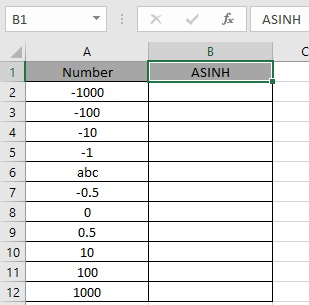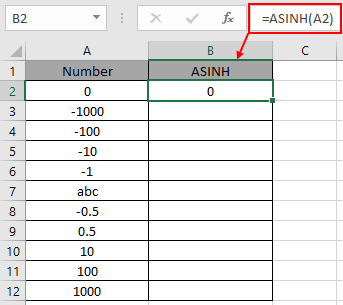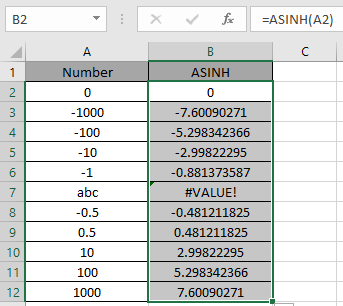# How to use the ASINH Function in Excel

SINH function is a Mathematical function to calculate the hyperbolic sine of the value. SINH function is built in excel function. Hyperbolic function of SINH defined using the exponential function.

Sinh (x) = (ex- e-x) / 2

Where ex = exponential function , e = 2.71828

So, it means Sine hyperbolic function returns the value as the formula stated above. Now if we wish to return the inverse of Sine hyperbolic function, excel ASINH function does our work. The below stated

x =  SINH -1(ex- e-x) / 2    { in mathematics }

x =  ASINH [(ex- e-x) / 2]   { in Excel }

So, The inverse hyperbolic sine is the value whose hyperbolic sine is the number, so ASINH(SINH(number)) equals number.

ASINH ( SINH ( number ) ) = number

ASINH function in excel returns inverse of the Sine hyperbolic function in excel. The

Syntax:

= ASINH ( number )

number : input any real number
Let’s understand this function using it in an example.Here we have values which will be input to the function and we need to find the inverse of the hyperbolic sine value using the ASINH function in excel

Use the formula:

=SINH ( A2 )

A2 : number given as cell reference.Here the argument to the function is provided as cell reference. As shown in the above snapshot, the Resultant comes out to be 0.

The value will calculated  using the mathematical formulation stated :

x = SINH -1 (e0- e0) / 2  = SINH -1( 1 - 1 ) / 2 = SINH -10 = 0

Now copy the formula to other cells using the Ctrl + D shortcut key.Excel calculates the inverse of the Hyperbolic sine of a number using the SINH function.

Here are some of the observations gathered using the ASINH function

Note:

1. The function returns #VALUE! Error if the argument to the function is non - numeric.
2. The input argument to the function can be any real number.
3. Argument to the function can be provided directly or using the cell reference in excel.

As you can see, we can get the inverse of the Sine hyperbolic function using ASINH function.

Hope you understood How to use ASINH function in Excel. Explore more articles on Mathematical function like ACOS H function and ATANH function here. Please state your query in the comment box below.

Related Articles

How to use the SINH function in Excel

How to use the SIN function in Excel

How to use Wildcards in Excel

Popular Articles

Edit a dropdown list

If with conditional formatting

If with wildcards

Vlookup by date

Terms and Conditions of use

The applications/code on this site are distributed as is and without warranties or liability. In no event shall the owner of the copyrights, or the authors of the applications/code be liable for any loss of profit, any problems or any damage resulting from the use or evaluation of the applications/code.